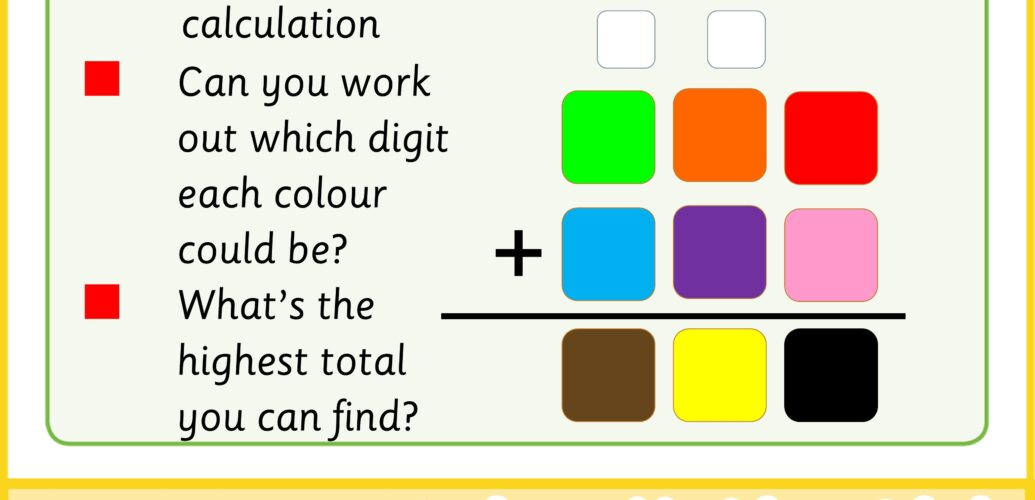# Colourithms

The puzzles this week are a form of cryptarithm. Cryptarithms use letters in place of digits to form a puzzle out of a standard arithmetical calculation. In this challenge, we’ll practice some of the techniques that should allow you to enjoy these puzzles. The beauty of colourithms is that they get children thinking about the techniques that we use for arithmetic rather than just following them.

Some of the related maths topics

• Long arithmetic
• Times tables
• Algebra
• Mental maths

To try this challenge: